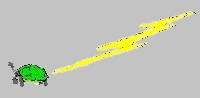# Usage of this WWW Site

Acknowledgements: A special thanks to Ben "VHDLCohen" for a number of these links.

## VHDL and Generators

 Arithmetic Module Generator for High Performance VLSI Designs Arithmetic Module Generator for High Performance VLSI Designs VHDL Library of Arithmetic Units VHDL Library of Arithmetic Units MICROSWISS Project TR-EZ-001 Reto Zimmermann Integrated Systems Laboratory Swiss Federal Institute of Technology (ETH) Zurich, Switzerland Distributed Arithmetic Abstract Distributed arithmetic is a bit level rearrangement of a multiply accumulate to hide the multiplications. It is a powerful technique for reducing the size of a parallel hardware multiply-accumulate that is well suited to FPGA designs. It can also be extended to other sum functions such as complex multiplies, fourier transforms and so on. Multiplication in FPGAs Abstract Multiplication is basically a shift add operation. There are, however, many variations on how to do it. Some are more suitable for FPGA use than others.  This page is a brief tutorial on multiplication hardware.

## Multiplication

 Distributed Arithmetic Abstract Distributed arithmetic is a bit level rearrangement of a multiply accumulate to hide the multiplications. It is a powerful technique for reducing the size of a parallel hardware multiply-accumulate that is well suited to FPGA designs. It can also be extended to other sum functions such as complex multiplies, fourier transforms and so on. Multiplication in FPGAs Abstract Multiplication is basically a shift add operation. There are, however, many variations on how to do it. Some are more suitable for FPGA use than others.  This page is a brief tutorial on multiplication hardware.

## Division

 LEON Division Hardware Divider For Leon Processor A European Space Agency have developed a processor named Leon. Synthesizable VHDL model of the processor is also made available. The project involves design and implementation of one of the following arithmetic units in two technologies; FPGA and Synopsys standard library. fp_divider.pdf A Floating Point Divider for RC Systems An Overview of Floating Point Arithmetic IEEE Floating Point Formats Examples of Floating Point Division Examples of Floating Point Addition Implementation of a 32-bit Floating Point Divider Conclusions http://www.eng.uci.edu/~alberto/PhDdiss/an99phd.pdf Low Power Division and Square RootAbstract The general objective of our work is to develop methods to reduce the energy consumption of arithmetic modules while maintaining the delay unchanged and keeping the increase in the area to a minimum. Here, we present techniques for dividers and square root units realized in CMOS technology. The energy dissipation reduction is carried out at different levels of abstraction: from the algorithm level down to the implementation, or gate, level. We describe the use of techniques such as switching-o not active blocks, retiming, dual voltage, and equalizing the paths to reduce glitches. Also, we describe modifications in the on-the- y conversion and rounding algorithm and in the redundant representation of the residual in order to reduce the energy dissipation. The techniques and modifications mentioned above are applied to several division and square root schemes, realized with static CMOS standard cells, for which a reduction in the energy dissipation of about 40 percent is obtained with respect to the standard implementation optimized for minimum delay. This reduction is expected to be even larger if low-voltage gates, for dual voltage implementation, are available. dh_arith_97.pdf SRT Division Architectures and ImplementationsSRT dividers are common in modern floating point units.  Higher division performance is achieved by retiring more quotient bits in each cycle. Previous research has shown that realistic stages are limited to radix-2 and radix-4.  Higher radix dividers are therefore formed by a combination of low-radix stages. In this paper, we present an analysis of the effects of radix-2 and radix-4 SRT divider architectures and circuit families on divider area and performance.  We show the performance and area results for a wide variety of divider architectures and implementations.  We conclude that divider performance is only weakly sensitive to reasonable choices of architecture but significantly improved by aggressive circuit techniques.  Lang  analyze the tradeoffs of using several of these optimizations in the context of static CMOS standard-cells.  Williams  presents a self-timed dynamic CMOS divider comprising a ring of five radix-2 stages that incorporates several of these techniques, and he also presents an analysis of the performance and area effects of the architectural components. Prabhu  presents the tradeoffs encountered when designing the Sun UltraSparc radix-8 divider.  In contrast to previous works, this paper analyzes in detail the effects of both circuit style and divider architecture on the area and performance of divider implementations.  We present the performance results using the technology-independent

## CORDIC

 The CORDIC Algorithmhttp://www.fpga-guru.com/files/crdcsrvy.pdf Summary CORDIC is an acronym for COrdinate Rotation DIgital Computer. It is a class of shift-add algorithms for rotating vectors in a plane. In a nutshell, the CORDIC rotator performs a rotation using a series of specific incremental rotation angles selected so that each is performed by a shift and add operation. Rotation of unit vectors provides us with a way to accurately compute trig functions, as well as a mechanism for computing the magnitude and phase angle of an input vector. Vector rotation is also useful in a host of DSP applications including modulation and Fourier Transforms. FPGA Implementation of Sine and Cosine Generators Using the CORDIC Algorithm Tanya Vladimirova Hans Tiggeler Surrey Space Center Military and Aerospace Applications of Programmable Devices and Technologies International Conference September 28-30, 1999 A2_Vladimirova_P.pdf A2_Vladimirova_P.doc Abstract This paper is concerned with FPGA implementation of CORDIC schemes for fast and silicon area efficient computation of the sine and cosine functions. The results of theoretical investigation into redundant CORDIC are presented. Summary of CORDIC synthesis results based on Actel and XILINX FPGAs is given. Finally applications of CORDIC sine and cosine generators in small satellites are discussed.Keywords CORDIC, sine, cosine, FPGA, synthesis, redundant signed-digit system.

Home
Last Revised: February 03, 2010
Digital Engineering Institute
Web Grunt: Richard Katz